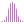#Variance (PQL)

Returns the statistical sample variance amount across elements in the specified list.

#### Syntax

Variance( <List> , OPTIONAL )

###### Syntax Alternatives

Var( <List> , OPTIONAL )

* Click on the function's arguments above for more details on the input values.

• There are 2 variants of the function: one for sample and one for the population ("P").
• List parameter should be a list or set of elements from an existing hierarchy in the model.
• The data point or tuple is optional, and describes the values used to generate the variance. If not supplied, the default measure or the query context will determine this value instead.
• For details on how to employ and use this function see the semantic calculation overview.
##### Different Function types
• This function is like the MDX variance function; it calculates the sample variance across a list of elements in a hierarchy or attribute.
• To use a simple variance mathematical operation on a list of numbers, use the Common library function. Use the square of standard deviation.

#### Examples

This example returns the sample variance expenses across the product category in the product table / dimension, using the sample demo model:

Var( {AllMembers([products].[Product Category])}, ([measures].[data Expenses]) )

Below, using the sample data of the 4 product categories we can use the above formula to calculate the sample variance. Looking at the list, the statistical variance value for expenses is 21,631,226,003,067 (which is standard deviation squared):